These Pages include various standards. To confirm the status of any standard, identify the replacement standard if it is obsolete and/or purchase the standard please use. BSI Shop It is also possible to become a BSI member and obtain copies of the Standards at much reduced prices.

Screws Index Page...

Joint Stiffness Notes

Introduction

To determine the performance of a bolted joint it is necessary to calculate the joint stiffness.  That is the deflection of the joint under a bolt loading condition When geometry of the bolted joint is an annulus with an OD less than 2,5 x the bolt diameter the joint stiffness can be conveniently calculated using k = EA/l .  When the joint includes a non-metallic gasket or material with a low modulus of elasticity then this item may have such a low stiffness value that the stiffness of the metal parts of the connection will have very low impact on the overall stiffness.

It is very difficult to obtain theoretical values of joint stiffness because a number of factors affect the result including.

 The effect of the angle of the screw thread Screw hole size Surface finish of contacting faces Flatness of contacting faces Use of Washer Selected size of contacting surface (d and D) Condition of surfaces between clamped plates

Any of these factors can have a significant effect on the resulting joint stiffness

The notes below identify methods which approximate the loading regime which has been found to occur by various studies using different methods.

Overall stiffness evaluation for multi-component joints

A bolted joint can include a number of separate parts.  The relationship between the individual stiffness values of the parts and the total stiffness of the clamped joint is as shown below..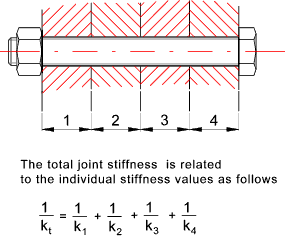The sketch below shows a bolted surface.  It has been shown using using ultrasonics and FEA that the pressure in a bolted surface is greatest under the bolt head and reduces as the distance from the bolt interface increases.  A method of be approximating the force distribution is based on use of the cone geometry as shown.  Various cone half-apex angles are used but for systems using a washer-face annulus and with hardened steel, cast iron or aluminium an angle of 25o to 35o considered reasonable.   These notes relate to half apex angle of 30o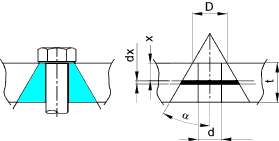Referring to the contraction of a small element dx of cone subject to a load P as shown.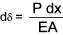The area is determined as follows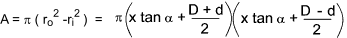Substituting this into the equation for contraction of an element..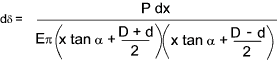To obtain the total deflection the equation above is integrated between 0 and t.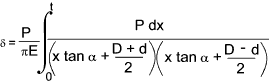Using integration manipulation techniques this results in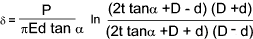The spring rate of the truncated cone is therefore ..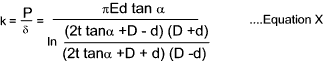The bolt hole diameter is d, the diameter of the applied pressure e.g.the washer diameter, is and the plate thickness is t.

For a half apex angle of 30o which provides a reasonable value for many engineering metals, the equation simplifies to..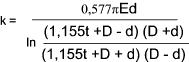Important Note: Widely different stiffness values result from different studies.  It has also been proved that different loading conditions and surface conditions also affect the resulting stiffness.

The variation in half apex angle α can be notice from the different studies completed.  Differences result from various reason including D/d ratios differences and surface finish difference

 Originator Technique Surface Type α Link 3 below Ultrasonics Ground 41o Link 3 below Ultrasonics Turned /Ground 68o Shigley and Mischke Analysis Any 30o Ito Ultrasonics Ground 70o Gould and Mikic Analysis Smooth 38o

Table A

Showing stiffness values for steel plate E = 207 kN/mm2 and a half apex angle α of 30 o calculated using equation X above..

d= bolt dia and D = 1,5 d = approximately as would result from a typical bolt or cap screw .

 - Plate Thickness (mm) 6 12 20 40 50 60 80 100 d D k = Stiffness (MN/mm ) based on...E = 207 kN/mm2 and α = 30o 6 9 2.757 2.102 1.83 1.619 1.576 1.547 1.511 1.489 8 12 4.245 3.098 2.622 2.254 2.178 2.127 2.063 2.024 10 15 6.009 4.236 3.503 2.934 2.817 2.739 2.639 2.579 12 18 8.047 5.514 4.47 3.66 3.493 3.381 3.239 3.153 16 24 12.945 8.49 6.661 5.245 4.953 4.757 4.508 4.356 20 30 18.932 12.017 9.191 7.006 6.556 6.253 5.868 5.634 24 36 26.008 16.095 12.055 8.94 8.3 7.867 7.32 6.986 30 45 38.658 23.236 16.974 12.163 11.175 10.509 9.664 9.149 36 54 53.75 31.605 22.638 15.768 14.36 13.41 12.207 11.474

Table B

Showing stiffness values for steel plate E = 207 kN/mm2 and a half apex angle α of 60 o calculated using Equation X above.  This table is provided to identify the variation is stiffness using two different, but still relevant, values of α

d= bolt dia and D = 1,5 d = approx as would result from a typical bolt or cap screw .

 - Plate Thickness (mm) 6 12 20 40 50 60 80 100 d D k = Stiffness (MN/mm ) based on...E = 207 kN/mm2 and α = 60o 6 9 5.627 4.929 4.642 4.423 4.378 4.349 4.312 4.289 8 12 8.108 6.886 6.382 5.995 5.917 5.864 5.798 5.759 10 15 10.88 8.996 8.216 7.616 7.494 7.412 7.31 7.248 12 18 13.939 11.255 10.142 9.284 9.11 8.993 8.845 8.757 16 24 20.909 16.216 14.27 12.764 12.456 12.25 11.99 11.833 20 30 29 21.76 18.758 16.431 15.955 15.635 15.231 14.988 24 36 38.203 27.878 23.602 20.284 19.604 19.146 18.569 18.219 30 45 54.079 38.121 31.526 26.407 25.355 24.647 23.753 23.211 36 54 72.426 49.629 40.231 32.938 31.437 30.426 29.148 28.373

Bolted joint comprising two equal plates

The force distribution on a typical bolted joint comprising two plates is shown below: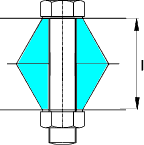It is necessary to obtain a value for the stiffness for each part of the joint.  In the case above for each of the two plates.

If the plates are of the same material and similar thickness then the overall stiffness of the joint is..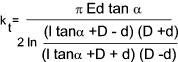FEA work has been completed (Wileman ,Choudury & Green)for similar 2 part bolted joint with typical washers (Dw = 1,5d) and joint members of the same material and an approximate curve fit curve for the resulting relationship has been derived..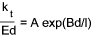Values for A and B are provided in the following table...

 Poisson Ratio Youngs Modulus A B Material GPa Steel 0,291 207 0,78715 0,62873 Aluminium 0,334 71 0,79670 0,63816 Copper 0,326 119 0,79568 0,63553 Gray Cast Iron 0,211 100 0,77871 0,61616 General Case 0,78952 0,62914

Example comparing stiffness value using two methods.

Consider two plates 20mm thick clamped with a 10m bolt using the table with a half apex angle α of 30 o

k for each plate from Table A above = 3 503 kN/mm .... 1/ k t = 1/k + 1/k .
Therefore k t = k/2 = 1 7513 kN/mm.

Using the formula above with A = 0,78715,B = 0,62873,d = 10, l=40 and E = 207 OOO
k t = E.d.A exp (Bd/l) = 1 906 kN/mm

 Sites Providing Information On Bolt Loading Engineers edge bolt preload calculator ..A very easy to use calculator for bolted flanges -very useful and convenient DAN_notes Loads in an elastic bolted assembly..Very detailed notes on bolt loading-similar to notes above. ExcelCalcs.com calculation Detailed Bolted Joint Calculations.xls..An exellent exel based worksheet showing all equations used

Screws Index Page...